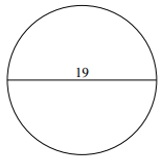# Circumference of a Circle

The circumference is the perimeter of a circle. It's the distance around a circle.Formula for Circumference of a Circle

• Circumference is given by the formula C = πd where π = 3.14 and d is the diameter of the circle.

• Circumference is given by the formula C = 2πr where π = 3.14 and r is the radius of the circle.

Find the circumference of following circle. Take π = 3.14### Solution

Step 1:

Given radius r = 7.5 units

Step 2:

Circumference of Circle = 2πr = 2(3.14)7.5 = 47.1 units

Find the circumference of following circle. Take π = 3.14### Solution

Step 1:

Given diameter = 19; radius r = 19/2 = 9.5 units

Step 2:

Circumference of Circle = 2πr = 2(3.14)9.5 = 59.66 units